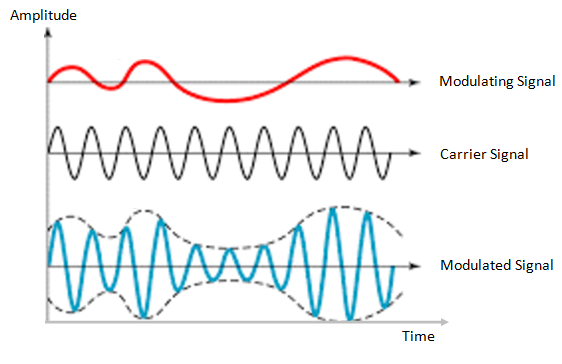# What Is Amplitude Modulation

## Amplitude Modulation

Amplitude Modulation may be defined as a system in which the maximum amplitude of the carrier wave is proportional to the instantaneous value (amplitude) of the modulating or base band signal.

Let us consider a sinusoidal carrier wave c(t) given as :

C(t) = A cos ωct         ……………….(1)

Here A is the maximum amplitude of the carrier wave and ωc is the carrier frequency. For simplicity here we have assumed that the phase of the carrier wave is zero in equation (1).

Let x(t) denote the modulating or base band signal.

Then according to amplitude modulation, the maximum amplitude A of the carrier will have to be made proportional to the instantaneous amplitude of the modulating signal x(t).

The standard equation for amplitude modulated (AM) wave may be expressed as :

s(t) = x(t) cos ωct + A cos ωct   ……….(2)

Or,

s(t) = [A + x(t)] cos ωct       ………………(3)

Figure 1 shows the modulating signal or base band signal, carrier signal and modulated signal.Fig 1

1. It may be observed that equation (1) or (2) describe the time-domain behaviour of amplitude modulated signal.
2. It may be noted that carrier signal [i.e c(t) =  A cos ωCt ] is a fixed frequency signal having frequency ωc .
3. The modulating or baseband signal x(t) contains the information or intelligence to be transmitted.
4. In the process of amplitude modulation, this information is super imposed upon the carrier signal in the form of amplitude variations of the carrier signal. In other words, in amplitude modulation, the information is transmitted in the form of amplitude variations of the carrier signal.
5. The resulting signal from the process of amplitude-modulation is called amplitude modulation signal of simply AM wave.
6. In the process of amplitude modulation, the frequency and phase of the carrier remain constant whereas the maximum amplitude varies according to the instantaneous value of the information signal.
7. Equation (3) represents an amplitude modulated wave. This wave has a constant frequency cos ωct and amplitude A + x(t) . This implies that the amplitude of the wave is changing around A in accordance with the value of the modulating signal x(t). The frequency of the AM signal remains unchanged and is equal to ωc
8. The AM wave has time-varying amplitude called as the envelope of the AM wave. Figure 1 shows that the envelope of AM wave consists of the modulating or baseband signal. This means that the unique property of AM is that the envelope of the modulated carrier has the same shape as the message signal or baseband signal.

We know that the expression for AM wave is  :

s(t) = [A + x(t)] cos ωct

Or,

s(t) =E(t) cos ωct       …………………(4)

Where,

E(t) = A + x(t)

E(t) is called the envelope of AM wave. This envelope consists of the baseband signal x(t). Hence, the modulating or baseband signal may be recovered from an AM wave by detecting the envelope.